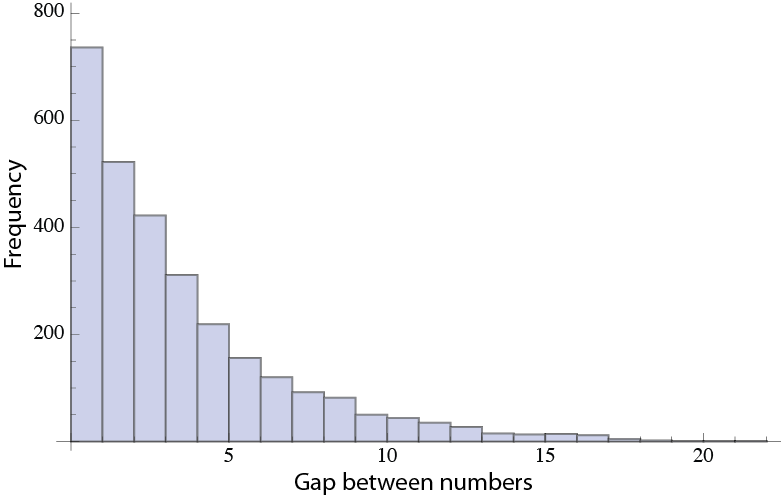# NMR, Compressed Sensing and sampling randomness Part II

##### Using Compressed Sensing: Selecting which points to collect.

NMR data is collected as a vector or matrix of points (depending how many dimensions the experiment has). Compressed sensing permits us to collect a subset of the actual points that are usually required. The question is, how do you decide which points to collect.

TL;DR Summary: Compressed sensing (CS) allows us to sample a subset of the points normally required for an NMR spectrum. CS theory suggests we should sample these points randomly. Random sampling leads to problems with the distribution of the gaps between samples. There is a better solution for NMR data.

##### The problem of Random Sampling.

Formal Compressed Sensing (CS) theory says we should collect a random spread of points between 1 and the final point (N). We could make a schedule of points by constructing a “dart-throwing” algorithm that randomly selects a point between 1 and N. If the algorithm selects a point twice, we just ask it to select another time. We run this until we have N/5 points. Surprisingly, it turns out this does not work well. Lets see why…

Below is an example of what happens when you select randomly 64 points out of 256 points. First of all, to make sure I selected points in an unbiased way, I took the numbers 1 through 256 into a vector and did a Fisher-Yates shuffle. Then I simply select the first 64 points in the vector after the shuffle. It has been shown that the Fisher-Yates shuffle is unbiased and I think it is a better solution to sampling purely randomly than a dart-throwing algorithm. Since I have 64 random points out of 256, you would expect that the average distance between numbers should be:

(256 – 64)/65 = 2.95385

This is because we need to eliminate 64 out of 256 (256 – 64) because they are selected and not part of gaps, then we must divide by 65 because by selecting 64 points we actually create 65 gaps. This means that the average gap size (that is the gap between selected numbers) is 2.95385.

I did 45 simulations like this, generating 64 * 45 = 2880 gaps. The average gap was 2.96319 so this basically looks good. Now, the Standard Deviation was 3.35334 which shows something funky is happening right away. This is a very large standard deviation for a mean value of 2.95385 and permits negative numbers to appear within one standard deviation. This can’t even happen for a gap as  gaps can’t be negative and there are no negative gap values in my numbers. How can this standard deviation be? Clearly the gaps are not normally distributed. Lets look at a histogram of the gap sizes.Yikes, its an exponential distribution. This has been noted before for random gap sizes, but I was amazed when I first saw this – in hind sight I’m not, but at first I was a little shocked. To confirm that this really does happen for random sampling I went looking for another example of a subset of random numbers selected from a pool of numbers. Lottery results of course are a good example.

I acquired the complete set of Megamillions lottery numbers from about June 2005 to October 2013 (the nature of the pool of numbers did not change in that time) and calculated the gaps between these random numbers. In this game you had to pick 5 numbers from 1-56 (the rules have since changed). So the average distance between numbers is

(56 -5) / 6 = 8.5

My calculated gap distance is 8.42009 and the histogram of the gap sizes is below.So, why does this matter? Well what is happening is the gaps are distributed in a way which can cause problems when we try and fill in the data we skipped in the gaps. To begin with look at the above histograms. When we randomly select points we end up making gaps such that the most common gap size is actually no gap at all. Thats pretty remarkable when you think about it. Then the second most common gap is the smallest gap, a gap of 1. By the time we have reach the average gap (around 8 for Megamillions, or a little less than 3 for selecting 64 points from 256) one half of our gaps have been accounted for, by definition. The reason so many gaps fall here is because there is a lower limit to the size of the gap. They can’t be less than zero. Now, all these small gaps are great, because they should be easy to fill in. The problem is the upper limit on the gap size is the size of the sampling space minus the number of samples. This number is always going to be several times larger than the average gap size. Simply put, this will always give the exponential distribution seen in the histograms, resulting in some gaps that are much larger than the average gap size. These large gaps present problems when trying to fill in data with predictive methods.

So how do we do better than this when ‘randomly’ selecting which samples to take? First of all, we don’t use a straight random algorithm – it makes gaps that are too large. Instead we introduced some randomness around the average gap size by using a poisson distribution. We call this Poisson Gap sampling, a method developed by Sven Hyberts in my lab.

Details will be in the next post.

This site uses Akismet to reduce spam. Learn how your comment data is processed.

Top